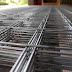Most Common Questions From Strength of Materials-

In civil engineering strength of materials is the core for advanced structure, RCC design etc.Here,we shared some hand-picked and top rated questions from strength of materials.

strength of material is one of the basic fundamental subject for civil engineering.It is considered as the base of civil engineering.when we talk about structural analysis,RCC design the concept of strength of material is most needed to solve many problem or to learn further details about structure.

Here i am giving you some hand picked useful questions and answers about strength of materials.

List Of Basic Fundamental Questions and Answers of Strength Of Materials-

1.Discuss the relationship b/w Ultimate stress and Working stress.

-Ultimate stress is basically the max amount of load that can be applied on a member without any failure.
Working stress is a type of stress that can be allowed for design purpose.

[Ultimate stress=Factor of safety* working stress]

[Note:while designing any member you have to ensure that working stress is not exceeding the ultimate stress]

2."Strain causes stress".justify the statement.

-To justify the above statement,lets take an example to clearly solve this puzzle.

suppose you are applying some external force to an object which will cause deformation(change in shape/strain) of the object.after that the object will try to reach its original shape by resisting that external force which you have applied.

In this case this internal force which will resist your external force is called 'stress'.

[object-external force-strain(deformation)-stress(resisting force)]. Thus "strain causes stress".

3.Explain the term "Poisson's ratio" ?

-Basically it is the ratio of lateral strain to longitudinal strain of a any member.
[longitudinal strain-Strain caused in the direction of application of load]

[lateral strain-Strain caused in the perpendicular direction of load]

For most of the metals the value of Poisson's ratio is 0.3.

4.What are the factors that affect the 'deflection of beam'?

- i.cross section of beam
ii.Young's modulus,
iii.Bending moment,
iv.length of beam,

5.What is 'Slenderness Ratio'?Give the value of slenderness ratio for long and short column.

-slenderness ratio=(effective length/least lateral dimension).

slenderness ratio for long column is >12,
slenderness ratio for short column is <12.

6.Why do we need 'Free-body-diagram' ?

- Free body diagram is basically a figure which indicates the magnitude and directions of all forces that are acting upon an object in a particular condition.

This diagram helps to visualise the nature of all forces acting upon an object which will further helps to calculate magnitude of forces,direction of forces etc.

7.Explain the limitation of 'Hook's Law' ?

-The main thing is this law is applicable only to those materials which are perfectly elastic.

Beyond the elastic limit this law fails.

[elastic limit:it is a point after which an object is being stretched becomes permanently stretched].

I hope you liked the basic questions about 'strength of materials'.

Want to know some top rated interview questions for civil engineering,

visit:

civil engineering interview questions

Basic civil engineering knowledge.

Most Common Questions From Strength of MaterialsReviewed by Civil049seminar on August 01, 2018 Rating: 5